## Algebra

Showing posts with label one-to-one. Show all posts
Showing posts with label one-to-one. Show all posts

### Inverse Functions

We begin with the definition: Inverse Functions – The functions f(x) and g(x) are inverses if bothfor all x in the domain g and f respectively.  In other words, if you compose inverse functions the result will be x.

Verify that the given functions are inverses.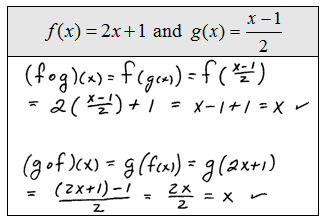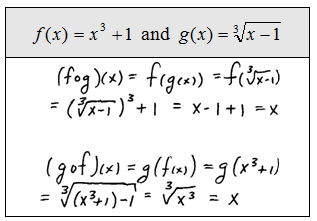When verifying that two functions are inverses, you must obtain the original value x by composing both ways.

Determine whether or not the given functions are inverses.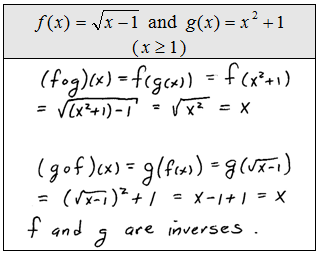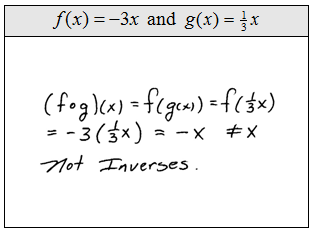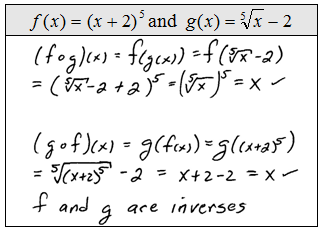If f and g are inverse functions, then g can be written using the notationwhich reads, "g equals f inverse." Caution: In this context, the -1 indicates an inverse function not a negative exponent.Take the time to review one-to-one (1-1) functions because it turns out that if a function is 1-1 then it has an inverse. Therefore, we may think of the horizontal line test as a test that determines if a function has an inverse or not.

Next we outline a procedure for actually finding inverse functions.

Step 1: Replace f(x) with y.
Step 2: Interchange x and y.
Step 3: Solve the resulting equation for y.
Step 4: Replace y with the notation for the inverse of f.
Step 5: (Optional) Verify that the functions are inverses.Find the inverse of the given function.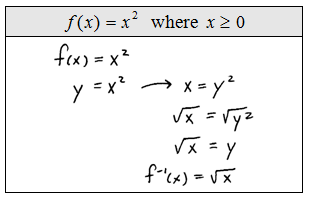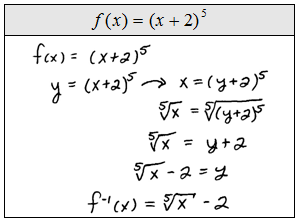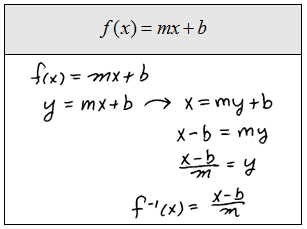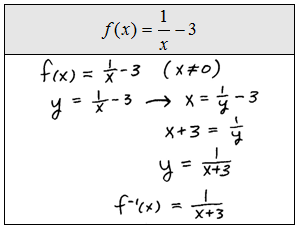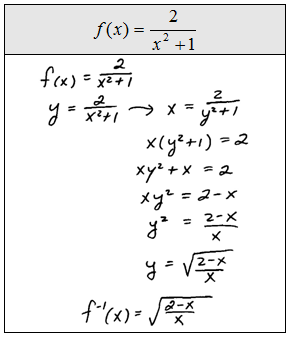Now that you know the definition of an inverse function and how to find them, we will next turn our attention to their graphs.  For any one-to-one function f whereand we have the following property.

Symmetry of Inverse Functions – If (a, b) is a point on the graph of a function f then (b, a) is a point on the graph of its inverse. Furthermore, the two graphs will be symmetric about the line y = x.

In the following graph, see that the functionshave symmetry when graphed on the same set of axes.Notice that (2, 3) is a point on f and (3, 2) is a point on its inverse. In other words, to graph the inverse all you need to do is switch the coordinates of each ordered pair. We used this fact to find inverses and will be very important in the next chapter when we develop the definition of the logarithm.

Given the graph of a 1-1 function, graph its inverse and the line of symmetry.